0
152

Download Pie Chart Questions for NMAT PDF. Top 10 very important Pie Chart Questions for NMAT based on asked questions in previous exam papers.

Take NMAT mock test

Instructions

Information: The graph below shows the number of subscribers of the Indian Ultimate Frisbee Association in each year since 2006. Membership was open to men from the year 2000 and to women also since the year 2008. Every year, 25% of the subscribers (i.e 25% of men and 25% of women) drop out and new members join in.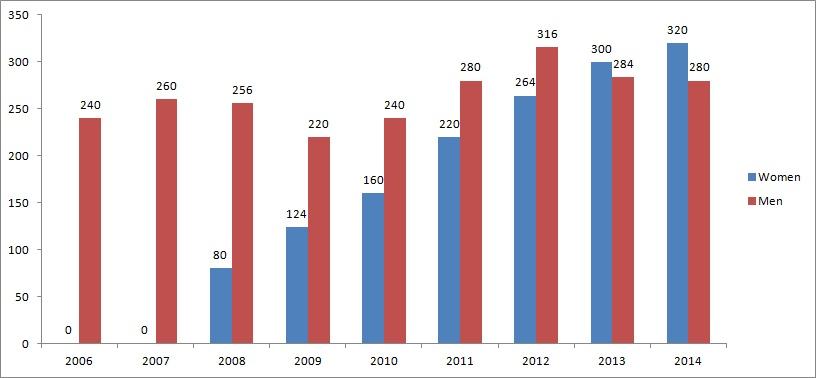Question 1: How many new female subscribers joined the Association in 2012?

a) 44

b) 55

c) 66

d) 99

Instructions

Information: The graph below shows the number of subscribers of the Indian Ultimate Frisbee Association in each year since 2006. Membership was open to men from the year 2000 and to women also since the year 2008. Every year, 25% of the subscribers (i.e 25% of men and 25% of women) drop out and new members join in.Question 2: How many new female subscribers joined the Association in 2012?

a) 44

b) 55

c) 66

d) 99

Instructions

Information: The graph below shows the number of subscribers of the Indian Ultimate Frisbee Association in each year since 2006. Membership was open to men from the year 2000 and to women also since the year 2008. Every year, 25% of the subscribers (i.e 25% of men and 25% of women) drop out and new members join in.Question 3: How many new female subscribers joined the Association in 2012?

a) 44

b) 55

c) 66

d) 99

e) tt

Instructions

The distribution of the availability of various mineral ores is as shown in the pie-chart. The distribution of Aluminium ores is further divided as shown in the second pie-chart.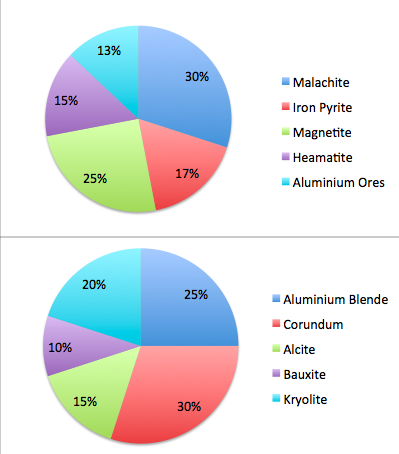Question 4: The pie-chart 2 showing the distribution of various aluminium ores were found to be erroneous. If the percentage of Aluminium Blende is 28% and all the other ores decrease proportionately, what is the angle of the ore Conundrum in pie-chart 2 ( rounded up to the nearest integer )?

a) 104 degrees

b) 99 degrees

c) 101 degrees

d) 105 degrees

e) 108 degrees

Question 5: The production cost of mining the various ores is as follows:
All Aluminium Ores: 20 crores per hectare
Iron pyrite: 15 crores per hectare
Magnetite: 10 crores per hectare
Malachite: 8 crores per hectare
Heamatite: 17 crores per hectare
For a company which is looking to spend the least amount of money, which of the following ores is the best to bid for ( The company which wins the bid for an ore has to mine it fully )?

a) Iron pyrite

b) Heamatite

c) Malachite

d) Either a) or b)

e) Either b) or c)

Question 6: The growth of some of the minerals in the following year is as follows:
Corundum : 600% ( due to the discovery of a new mine of Aluminium )
Kryolite: 900%
Malachite: -12%
Heamatite: 3%
Magnatite : 5%
Then which of the following ores has the highest absolute value in terms of availability next year?

a) Magnetite

b) Malachite

c) Corundum

d) Kryolite

e) Data Insufficient

Instructions

A school band has 2 trumpeteers, 5 violinists, 3 pianists and 6 drummers at the start of the school year in July 2007. For the next four years, the band adds a member to it on 31st December, assigning the new recruit to one of the four groups. At the time of joining the band, each of the new recruit is 9 years old.
Over the four years each group got one new member. Also, one of the band members graduated from the school in these four years and hence left the band at the age of 17. The graph shows a groupwise average age over the four years 2007-2010 as measured on 31 December after the changes to the band.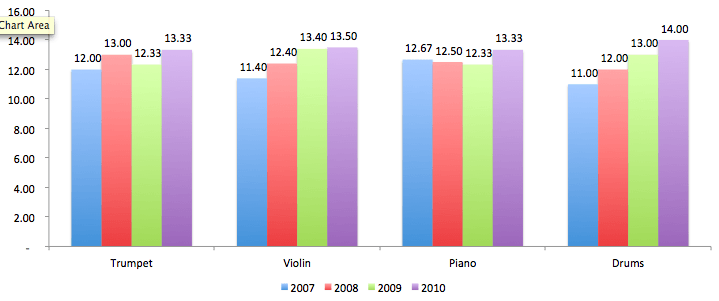Question 7: What was the age of the new recruit who joined the trumpet section as on 31st Dec 2010?

a) 10

b) 11

c) 12

d) 13

e) Can’t be determined

Question 8: If one of the members from the Piano group was aged 12 on 31/12/2007 then what was the age of the youngest group member on 31/12/2007?

a) 11

b) 12

c) 10

d) 14

e) Insufficient data

Question 9: The band member who left the band played which instrument?

a) Trumpet

b) Violin

c) Piano

d) Drums

e) Can not be determined

Instructions

The bar chart shows the number of cars made by companies A and B in the years 2005 to 2010. Answer the questions based on the data.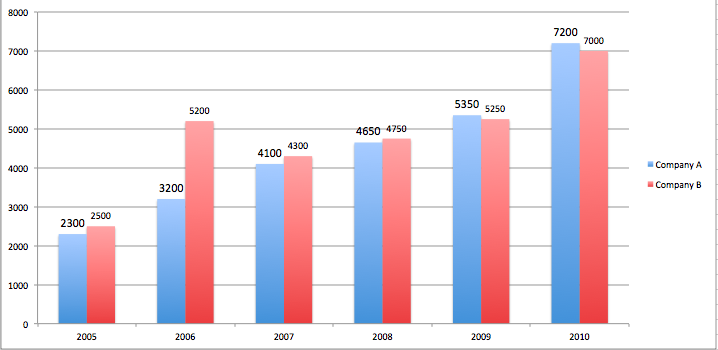Question 10: If company B exhibits the same cumulative annual growth rate, then approximately how many cars will be produced by company B in the year 2011?

a) 7600

b) 8600

c) 7280

d) 8940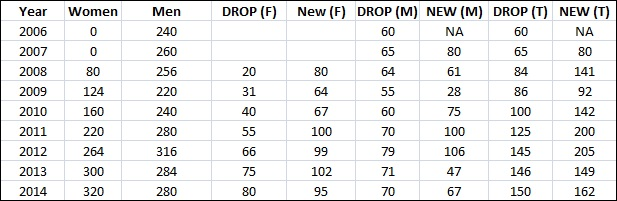The number of female subscribers in 2011 is 220.
So, number of female subscribers who quit is 220/4 = 55.
Hence, the new female subscribers in the year 2012 is 264-220+55 = 99The number of female subscribers in 2011 is 220.
So, a number of female subscribers who quit is 220/4 = 55.
Hence, the new female subscribers in the year 2012 is 264-220+55 = 99The number of female subscribers in 2011 is 220.
So, a number of female subscribers who quit is 220/4 = 55.
Hence, the new female subscribers in the year 2012 is 264-220+55 = 99

Percentage of Conundrum = 30%.
It has to share the loss of 3% proportionately with the others, which form a total of 75%.
Therefore, loss in percentage of Conundrum = (30/75)*3% = 1.2%.
Therefore, new percentage of conundrum = 28.8%.
The angle = (28.8/100)*360 = 103.68 degrees.
Therefore, answer is 104 degrees ( rounded-up ).

The total cost of mining the ores is as follows:

Aluminium Ores: 20*13k = 260k
Iron pyrite : 17*15k = 255k
Magnetite: 10*25k = 250K
Malachite: 8*30k = 240k
Heamatite: 15*17k = 255k

Therefore, the least production cost is for Malachite.

Let the total availability of all ores be 100 units.

Hence, initially the values for each of the ores is

Corundum: 30% of 13% of 100 = 3.9

Malachite: 30% of 100 = 30

Magnatite: 25% of 100 = 25

Kryolite: 20% of 13% of 100 = 2.6

According to the given information, the values of the ores after the growth would be as follows:

Corundum: 3.9 * (1+600%) = 27.3

Malachite: 30 * (1-12%) = 26.4

Magnatite: 25 * (1+5%) = 26.25

Kryolite: 2.6* (1+900%) = 26

Therefore, the absolute value of Corundum would be the highest.

If there are no additions/deletions in a group, the average should increase by 1 year.
As Drums group shows no changes between Dec 2007-Dec 2010, the new recruit into Drums would have joined on Dec 2007.
In the Trumpets group, the average changes from 13 to 12.33 suggesting the latter average is calculated by dividing by a multiple of 3.
Hence, there was an addition to the Trumpet group in 2009.
Violin group, the average changes from 13.4 to 13.5 over the last year indicating the divisor is now either 4 or 6.
An average of 13.4 indicates the divisor is 5.
Hence the sum of the ages changes from 67 to either 54 or 81.
If a 17 yr old member had left then the change would have been 67-17+4=54.
If a 9 yr old member had been added the change would have been 67+5+9=81.
In the Piano group, the average changes from 12.67 to 12.5.
Hence the number of members has changed from 3 to either 2 or 4.
Hence sum of ages = 38 changes to either 25 or 50.
If the change was due to the graduation of members it should have changed to 38-17+2=23.
Therefore, it cannot be a deletion. Hence a member is added in 2008.
Hence the change in 2009 has to be a deletion due to graduation.
Thus, a member was added to Violin in 2010.
As per the explanation, a new recruit was added to the Trumpets section in 2009.
Hence, his/her age in 2010 would be = 9+1 = 10 years

If there are no additions/deletions in a group, the average should increase by 1 year.
As Drums group shows no changes between Dec 2007-Dec 2010, the new recruit into Drums would have joined on Dec 2007.
In the Trumpets group, the average changes from 13 to 12.33 suggesting the latter average is calculated by dividing by a multiple of 3.
Hence, there was an addition to the Trumpet group in 2009.
Violin group, the average changes from 13.4 to 13.5 over the last year indicating the divisor is now either 4 or 6.
An average of 13.4 indicates the divisor is 5.
Hence the sum of the ages changes from 67 to either 54 or 81.
If a 17 yr old member had left then the change would have been 67-17+4=54.
If a 9 yr old member had been added the change would have been 67+5+9=81.
In the Piano group, the average changes from 12.67 to 12.5.
Hence the number of members has changed from 3 to either 2 or 4.
Hence sum of ages = 38 changes to either 25 or 50.
If the change was due to the graduation of members it should have changed to 38-17+2=23.
Therefore, it cannot be a deletion. So, a member is added in 2008.
Hence the change in 2009 has to be a deletion due to graduation.
Thus, a member was added to Violin in 2010.
As per the explanation, a member was added to the Piano group in 2008 and a member graduated from the group in 2009 at the age of 17.
In 2009, the sum of the ages hence was 12.33*3=37 = 10+x+y.
Hence x+y=27.
If one of the members was 12 in 2007, they would be 14 in 2009.
Hence y=27-14=13.
Hence the youngest member was 13-2=11 years old.

If there are no additions/deletions in a group, the average should increase by 1 year.
As Drums group shows no changes between Dec 2007-Dec 2010, the new recruit into Drums would have joined on Dec 2007.
In the Trumpets group, the average changes from 13 to 12.33 suggesting the latter average is calculated by dividing by a multiple of 3.
Hence, there was an addition to the Trumpet group in 2009. Violin group, the average changes from 13.4 to 13.5 over the last year indicating the divisor is now either 4 or 6.
An average of 13.4 indicates the divisor is 5.
Hence the sum of the ages changes from 67 to either 54 or 81.
If a 17 yr old member had left then the change would have been 67-17+4=54.
If a 9 yr old member had been added the change would have been 67+5+9=81.
In the Piano group, the average changes from 12.67 to 12.5.
Hence the number of members has changed from 3 to either 2 or 4.
Hence sum of ages = 38 changes to either 25 or 50.
If the change was due to the graduation of members it should have changed to 38-17+2=23.
Therefore, it cannot be a deletion. Hence a member is added in 2008.
Hence the change in 2009 has to be a deletion due to graduation.
Thus, a member was added to Violin in 2010.
As per the explanation, the member who left was from the Piano group